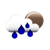### Percentage Calculator

 1. What is P% of X ? What is % of ? Answer:

 2. P is what percentage of Y ? is what percent of ? Answer: %

 3. Z is P percent of what ? is % of what ? Answer: %

In mathematics, a percentage is a number or ratio expressed as a fraction of 100. It is often denoted by the percent sign symbol: "%". It can also be denoted by 'percent'.65% is equivalent to the decimal 0.65.

In other words, if you want to turn a percentage into a decimal, just divide it by 100. For example, 65% = 65 ÷ 100 = 0.65. In reverse, to change a decimal into a percentage, you just need to multiply it by 100. For 0.65, the percentage is: 0.65 × 100 = 65%.

Besides, it is also equal to the fraction:

65 / 100

#### How to Calculate Percentages

You can use the percentage formula to find percentage of a number:

Percentage
=
Value / Total Value
× 100

Example: What is the percentage of 12 over 20 ?

Percentage
=
12 / 20
× 100

Anwser: 60%

To know how to find percentage of any numbers? Please use our percent calculator.

#### References

More references for Percentage

#### Local Weather Status

Ashburn, United States
9th December, 2019 Monday
Light Rain6.67 - 9.44
Humidity: 93 %
Wind: 4.6 km/h